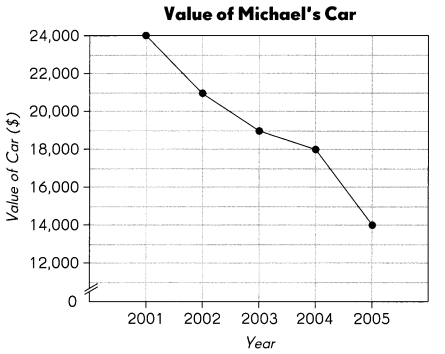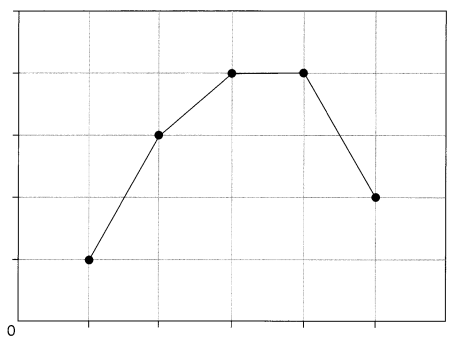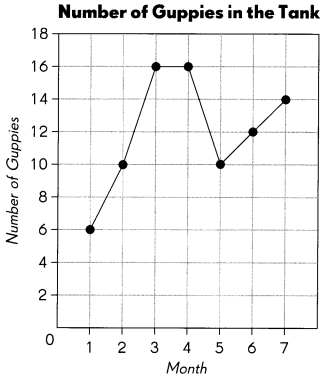# Math in Focus Grade 4 Chapter 4 Answer Key Tables and Line Graphs

Go through the Math in Focus Grade 4 Workbook Answer Key Chapter 4 Tables and Line Graphs to finish your assignments.

## Math in Focus Grade 4 Chapter 4 Answer Key Tables and Line Graphs

Math Journal

Michael bought a new car in 2001 for \$24,000. The line graph shows how the value of his car changed from 2001 to 2005.Write four questions that can be answered using the data in the line graph. Then write the answers.

a. Question
_____________
_____________
_____________
_____________

b. Question
_____________
_____________
_____________
_____________

c. Question
_____________
_____________
_____________
_____________

d. Question
_____________
_____________
_____________
_____________

Challenging Practice

Look at the line graph.Question 1.
Suggest what data this graph could be showing.

Question 2.
Create a title, scale and labels for the graph. Show these on the graph.

Question 3.
Why do you think the line is horizontal from point 3 to point 4?

Problem Solving

The graph shows the number of guppies in a tank over a few months.Question 1.
During which 1 -month interval was the increase in the number of guppies the greatest?